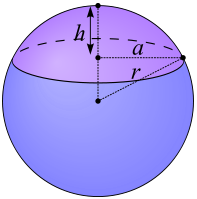## Sphere Cap Area Volume Calculator

 Radius (r): Sphere Cap Height (h): Sphere Cap Volume : 1  π Cap Surface Area : 1  π

In geometry, a spherical dome also called spherical cap is a portion of a sphere cut off by a plane.

### Sphere (Cap) Formulas1. Volume = 4 * π * r3 / 3

2. Surface Area = 4 * π * r2

3. Volume of Shaded Cap = π * h2 * (3r - h) / 3

4. Surface Area of Shaded cap = 2 * π * r * h

There is a sphere cap called as hemisphere, it is plane passes through the center of the sphere, the height of the cap is equal to the radius of the sphere.

Thinkcalculator.com provides you helpful and handy calculator resources.